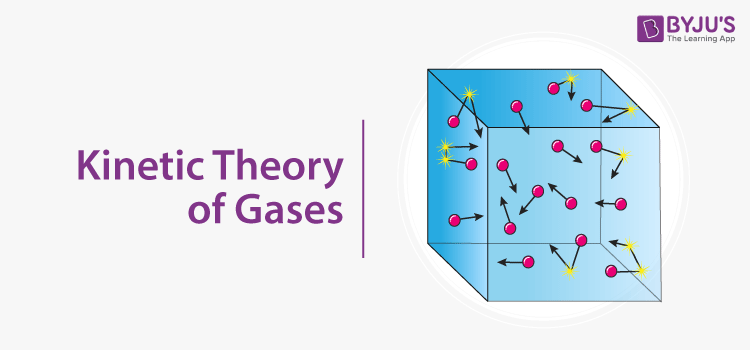Checkout JEE MAINS 2022 Question Paper Analysis : Checkout JEE MAINS 2022 Question Paper Analysis :

# What is the Kinetic Energy of a Gas

Kinetic Molecular Theory explains that particles of gas are in continuous motion and show perfectly elastic collisions. It is possible to use Kinetic Molecular Theory to describe all the Laws of Charles and Boyle. In a series of gas ions, the total kinetic energy is directly proportional to absolute temperature alone.

Kinetic energy is exactly proportional to the object’s mass and its velocity square: K.E. = 1/2 V2 m. If the mass has units of kilogrammes and a velocity of metres per second, the kinetic energy has units of kilogrammes per second of square metres. The simplest kinetic model is based on the assumption that the gas consists of a large number of similar molecules travelling in random directions, divided by large distances relative to their size.(0)(5)